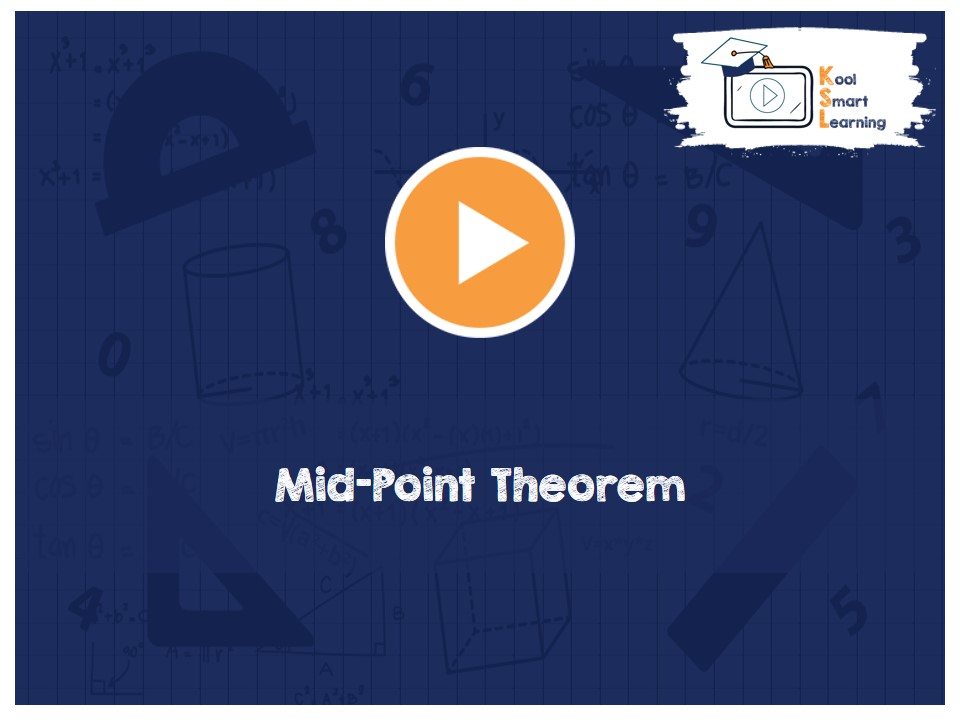At KoolSmartLearning, we intend to harness the power of online education to make learning easy.## Mid-Point Theorem

/  Mid-Point Theorem## Mid-Point Theorem

This video talks about mid-point theorem (The line segment joining the mid-points of two sides of a triangle is parallel to the third side) and its converse theorem (The line drawn through mid-point of one side of a triangle parallel to the another side bisects the third side). The proof of each one of the theorems is also explained in the video.

More Related Videos